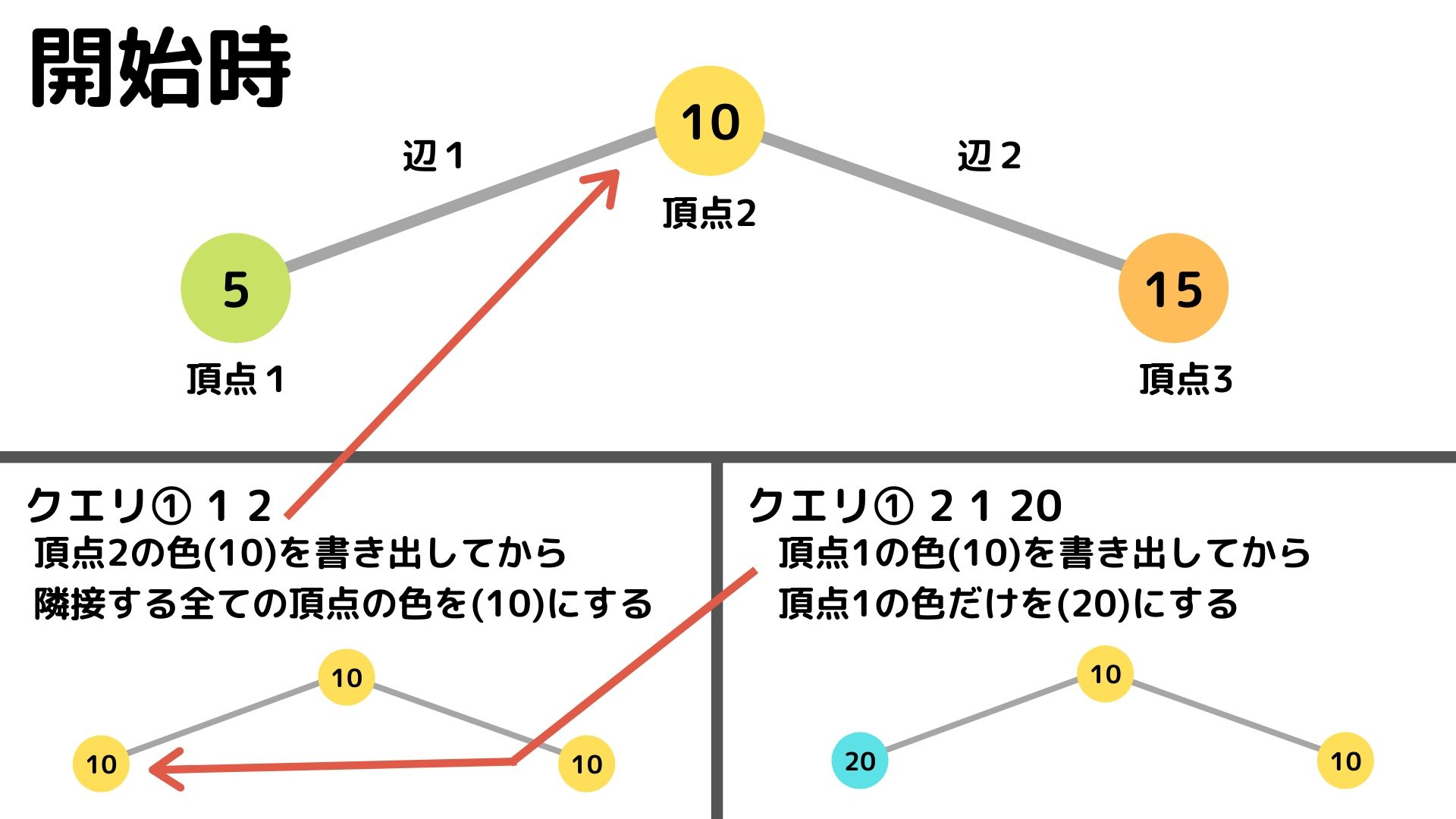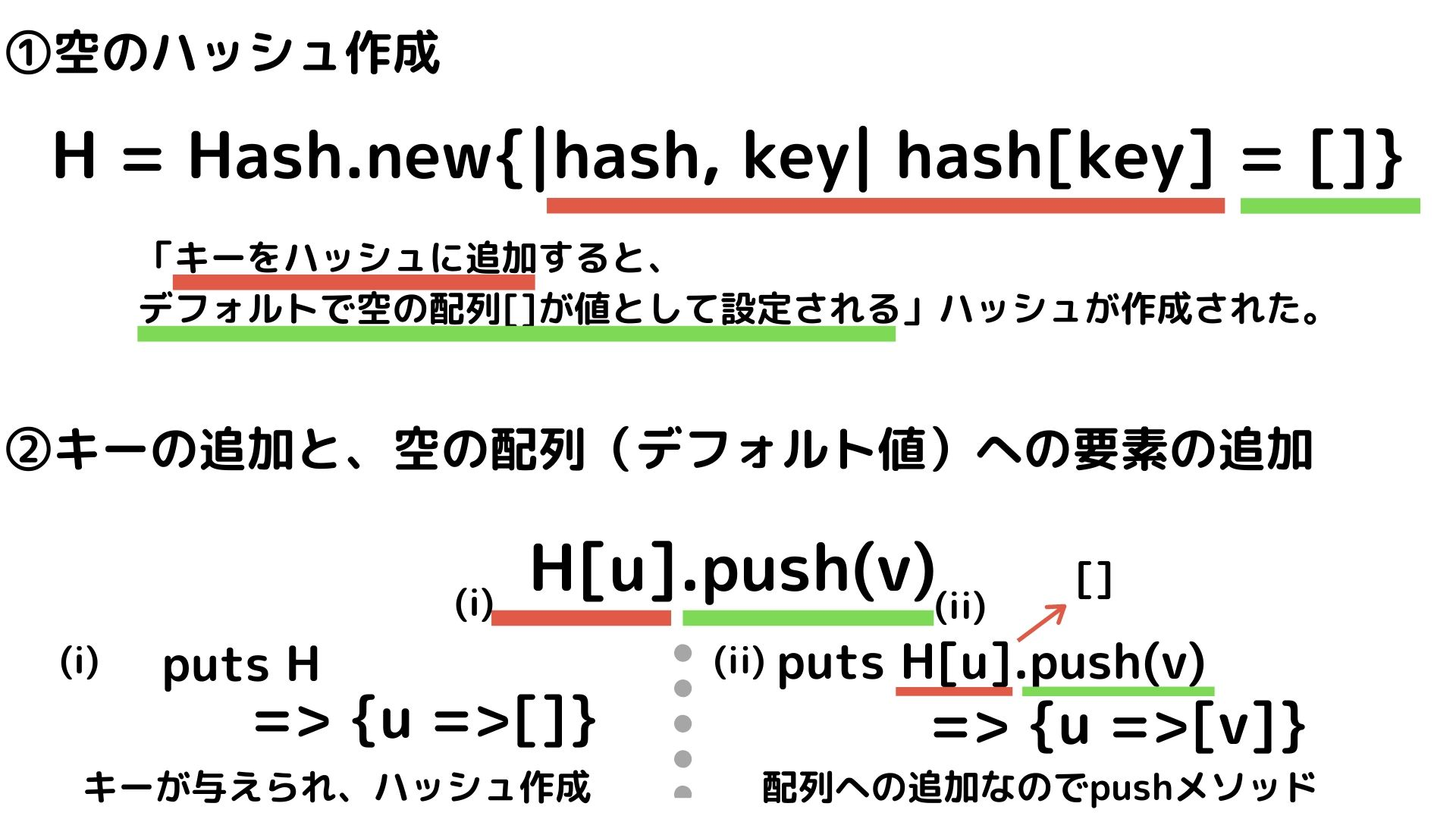# Learning Ruby with AtCoder 14 [3rd Algorithm Practical Test Sprinkler] Hash creation, key / value addition

## Introduction

Participating in competitive programming as part of learning Ruby and algorithms. Here, we output what we learned during learning.

This time about hashes. The reason for writing this article was the 3rd Algorithm Practical Test, 5th Question "Sprinkler".

This question was a question that could be answered smoothly using a hash, I had little understanding of hashes and couldn't answer this question during the test ...

So this time,

① Answer this question using a hash ② Think about how to use the hash based on the answer

With this kind of flow, I would like to summarize it in my own way.

## problem

An undirected graph consisting of N vertices numbered 1,2,3, ..., N and M undirected edges numbered 1,2,3, ..., M Given. Edge i connects the vertices ui and vi in both directions.

Each vertex can be colored, initially vertex i is painted with the color ci. (In this problem, colors are represented by integers greater than or equal to 1 and less than or equal to 10 ** 5).

Sprinklers are installed at each apex. When you launch the sprinkler at vertex i, The colors of all vertices adjacent to vertex i are repainted with the color of vertex i when the sprinkler is activated.

Process the Q queries s1, s2,…, sQ given in the following format in order. --Output the current color of vertex x. Then launch the sprinkler at vertex x. Given in the form 1 x. --Output the current color of vertex x. Then overwrite the color of vertex x with y. Given in the form 2 x y.

** Constraints ** --All given inputs are integers

• 1≤N,Q≤200
• 0≤M≤N(N−1)/2
• 1≤ui,vi≤N
• 1≤ci≤10**5 --i is a character string in one of the following formats
• '1 x'(1≤x≤N)
• '2 x y' (1≤x≤N,1≤y≤10**5) --There are no multiple edges or self-loops in a given graph

The input is given in the following form.

``````N M Q
u1 v1
⋮
uM vM
c1 c2 ⋯ cN
s1
⋮
sQ

Input example
3 2 3
1 2
2 3
5 10 15
1 2
2 1 20
1 1
``````
``````Output example
10
10
20
``````

The image of the problem looks like this:``````N, M, Q = gets.split(" ").map(&:to_i)

H = Hash.new{|hash, key| hash[key] = []}　#① Create an empty hash

M.times do
u, v = gets.split(" ").map(&:to_i)
end

C = gets.split(" ").map(&:to_i)
C.insert(0, nil)

Q.times do
x,y,z = gets.split(" ").map(&:to_i)
puts C[y]
if x == 1
H[y].each{|i| C[i]=C[y] }
else
C[y] = z
end
end
``````

### ① Create an empty hash

In the above solution, we prepare an empty hash by giving a block {} to the new method of the Hash class.

``````H = Hash.new{|hash, key| hash[key] = []}
``````

Each time you add a key and value to the hash, the block is evaluated and an object is created. In the code above, an empty array is the default value for the value. This is because the question we are answering this time is a question where there may be multiple values for one key. (The key contains one vertex, and the value contains the vertex adjacent to that vertex (connected by an edge))

The object received from the standard input is added to the hash as a key and value. In this answer, the value is added as an element to the array set as the default value.

``````u, v = gets.split(" ").map(&:to_i)
H[u].push(v) #u as the key and v as the value
#Since it is an addition to the array, "<<You can also use the "" symbol.
H[u] << v
``````

I tried to summarize the image so far in my own way.It's a little cluttered, but I feel like I've finally got the image.

If you want to use hashes (1) Set the relationship between the key and the value in the block. (2) Every time a key is added to the hash, a value is prepared according to (1). (3) When adding or changing the value, follow the default value.

For the time being, I found that I could tackle this problem in this way.

## Finally

So far, I have summarized the creation of hashes and the addition of keys and values in my own way. I want to use hashes more and more and make them my own.

I intend to summarize it while reading the reference etc. If you have any mistakes, I would be grateful if you could point them out.